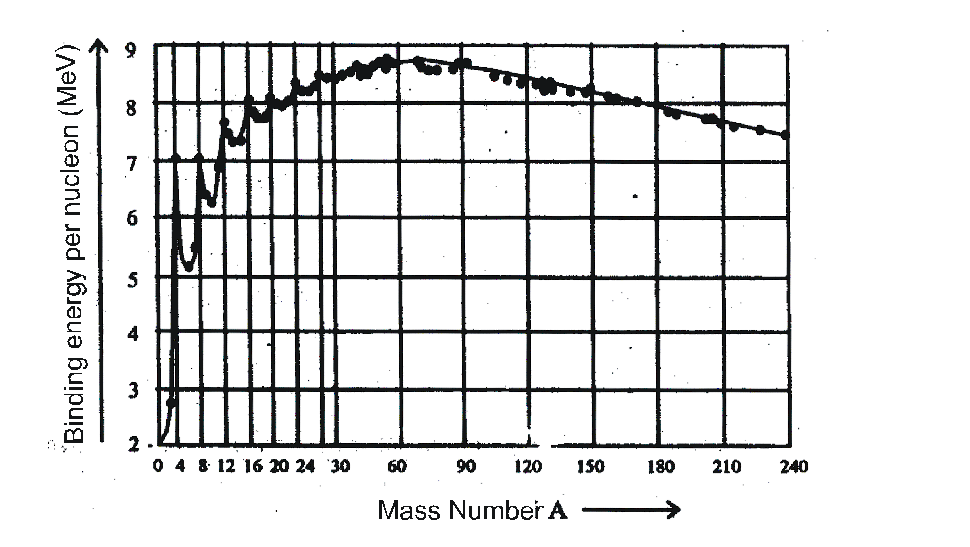Most Affordable JEE | NEET | 8,9,10 Preparation by Kota's Top IITian Doctor Faculties

# What is binding energy in physics - Binding energy per nucleon - eSaralHey, do you want to learn about What is binding energy in physics? If yes. Then keep reading.

## Binding energy

1. The energy required to break a nucleus into its constituent nucleons and place them at an infinite distance is called binding energy.

2. The energy equivalent to mass defect is called binding energy.

3. This is the energy with which the nucleons are held together.

4. The binding energies $(\sim \mathrm{MeV})$ are very large as compared to molecular binding energies

$(\sim \mathrm{eV})$

Binding energy

$B E=(\Delta m) c^{2}$

$=c^{2}\left[Z m_{p}+(A-Z) m_{n}-M\left(z X^{A}\right)\right]$

the rest mass of protons + rest mass of neutrons = rest mass of nucleus + BE

## Binding energy per nucleon

1. The binding energy per nucleon of a nucleus is the average energy required to extract a nucleon from the nucleus. Binding energy per nucleon

$\overline{\mathrm{B}}=\frac{\text { Total binding energy }}{\text { Total number of nucleons }}$

$=\frac{\mathrm{BE}}{\mathrm{A}}=\frac{\Delta \mathrm{mc}^{2}}{\mathrm{~A}}$

$=\frac{c^{2}}{A}$ x
$\left[Z m_{p}+(A-Z) m_{n}-M\left(z X^{A}\right)\right]$

2. The plot of binding energy per nucleon with mass number A is shown as3. Binding energy per nucleon gives a measure of the stability of the nucleus. More is the binding energy per nucleon more is the stability of the nucleus.

4. Binding energy per nucleon gives a measure of the stability of the nucleus. More is the binding energy per nucleon more is the stability of the nucleus.

5. Binding energy per nucleon is small for lighter nuclei i.e. ${ }_{1} \mathrm{H}^{1},{ }_{1} \mathrm{H}^{2}$ etc.

6. For A < 28 at A = 4n the curve shows some peaks at

${ }_{2} \mathrm{He}^{4},{ }_{4} \mathrm{Be}^{8},{ }_{6} \mathrm{C}^{16},{ }_{8} \mathrm{O}^{16},{ }_{10} \mathrm{Ne}^{20},{ }_{12} \mathrm{Mg}^{24}$.

7. The binding energy per nucleon is almost constant about 8.5 MeV in range 40 < A < 120. The binding energy per nucleon is maximum about 8.8 MeV for $\mathrm{F} \mathrm{e}^{56}$.

8. The binding energy per nucleon decreases for A > 200 They become less stable and exhibit radioactivity.

9. In fusion lighter nuclei fuse to form heavier nuclei. The process in accompanied by an increase in binding energy per nucleon.

10. In fission a heavy nucleus splits into two lighter nuclei. Here also increase in binding energy per nucleon takes place.

11. The heaviest stable nuclide is ${ }_{83} \mathrm{Bi}^{209}$.

so, that's all from this article. I hope you get the idea about What is binding energy in physics. If you found this article informative then please share it with your friends. If you have any confusion related to this topic then feel free to ask in the comments section down below.

For a better understanding of this chapter, please check the detailed notes of Chapter Nuclei. To watch Free Learning Videos on physics by Saransh Gupta sir Install the eSaral App.